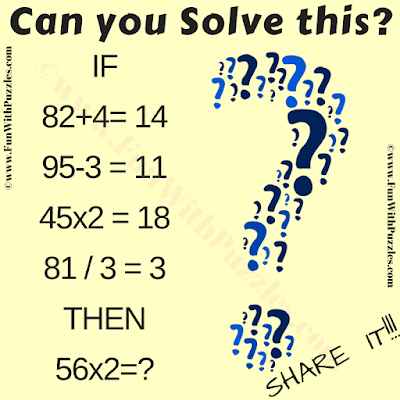This is very interesting "Crack the Code Riddle". This the combination of a logical reasoning riddle and math riddle. In this picture riddle, your first challenge is to crack the logical code in the given number equations. Once you are able to crack this code, then perform the mathematical calculations to get the value of the missing number which will replace the question mark in the last logical equation.Can you Crack the Code?
The answer to this "Crack the Code Math Riddle", can be viewed by clicking on the answer button.